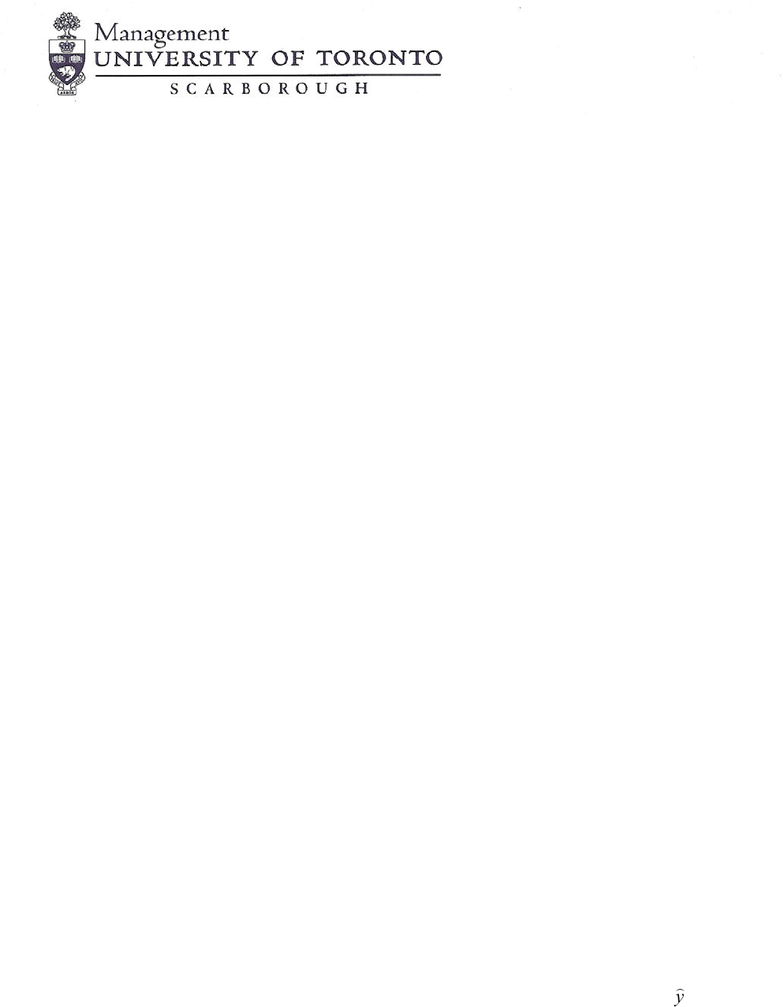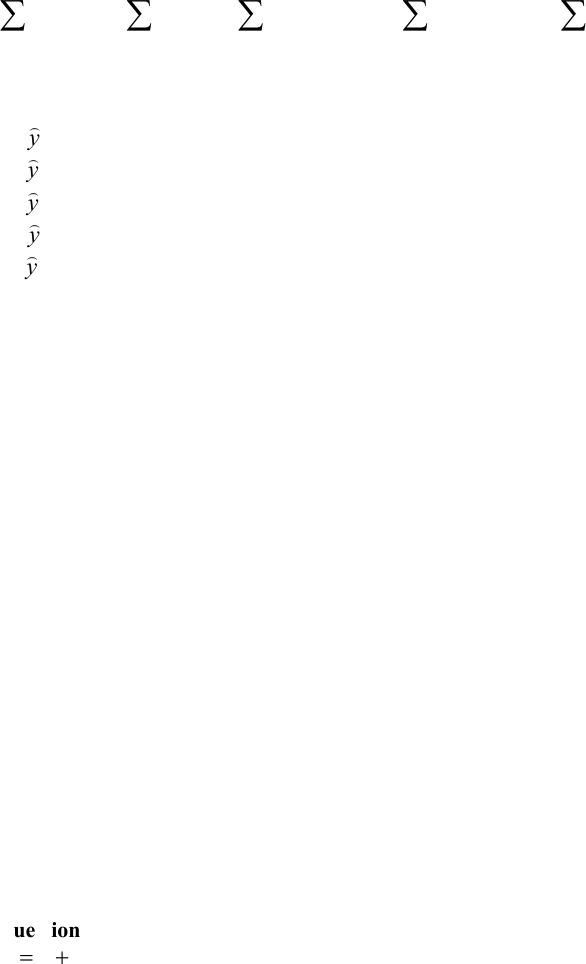Class Notes (1,100,000)
CA (650,000)
UTSC (30,000)
ATA (10)
Lecture 5

# MGEB12H3 Lecture Notes - Lecture 5: Simple Linear Regression, The Intercept, Sleep DeprivationExam

Department
Economics for Management Studies
Course Code
MGEB12H3
Professor
ATA
Lecture
5

This preview shows pages 1-3. to view the full 14 pages of the document.MGEB12: Quantitative Methods in Economics-II
Problem Set-5
Chapter 14: 2, 16, 18, 24, 26, 32,34, 36, 45, 46
Supplemental
1. When the least squares estimate of the slope, b1, is zero, the least squares estimate of the intercept, b0, is:
A. the mean of the independent variable,
B. the mean of the dependent variable,
C. the mean of the dependent and independent variables combined,
D. another complex function of the data,
E. None of the above.
2. In finding a regression line, the method is called least squares because:
A. we minimize the sum of the squared differences between the observed values of Y and the predicted values
of Y.
B. we minimize the sum of the squared differences between the observed values of Y and the mean of the
predicted value of Y.
C. we minimize the difference between the mean of the observed of Y and the predicted value of Y.
D. we minimize the sum of the differences between the observed values of Y and the predicted values of Y.
E. None of the above.
3. The error in the population linear regression model
A. accounts for variability in Y that is unexplained by X.
B. is observed only in the sample of observations that is collected.
C. has a variance that depends on the variance of the distribution of the intercept.
D. is equal to zero for observations in which observed X & Y are equal to its sample mean.
E. is equal to zero for the smallest observation.
4. In a simple linear regression, the slope is:
A. always equal to the correlation coefficient.
B. equal to the correlation coefficient only if r = +1.
C. equal to the correlation coefficient if r = -1.
D. equal to the correlation coefficient if the x and y variables are in standardized form.
E. not related to the correlation coefficient.
5. An OLS (simple regression) analysis is conducted and the fitted regression line is found to be
y
= 1 +
4.25x. The Excel output shows that SST = 2.0, SSR = 1.5, and SSE = 0.5. The percent of variation in y
explained by x in the OLS analysis is:
A. 100%
B. 50%

Only pages 1-3 are available for preview. Some parts have been intentionally blurred.2
C. 75%
D. 20%
E. not enough information is given to answer the question
6. Following a simple regression on 23 pair of observations you have estimated the slope parameter to be b1 = -
0.9225 with SE(b1) = 0.65. If you are interested to test if the true slope1) is equal to 0 against the alternative
that is less than zero, the most accurate bounds for the p-value would be:
A. 0.20 > p-value > 0.10
B. 0.10 > p-value > 0.05
C. 0.05 > p-value > 0.025
D. p-value < 0.025
E. p-value > 0.20
7. A least squares regression is conducted on 27 (x, y) pairs, resulting in an estimate b1 = 4.31 of the slope with
SE(b1) = 4.33. The most accurate bounds for the p-value for a test of the null hypothesis that the true slope β1 is
equal to zero versus the alternative that it is not equal to 0, is:
A. .10 < p-value < .20
B. .20 < p-value < .40
C. .05 < p-value < .10
D. p-value < :0005
E. .0005 < p-value < .005
8. A least squares regression is conducted on 27 (x,y) pairs, resulting in an estimate b1 = 2.23 of the slope with
SE(b1) = 1.31. The 99 percent confidence interval for the true slope β1.
A. ( -1.42; 5.88)
B. (2.078; 2.382)
C. (2.119; 2.341)
D. (-1.22; 5.68)
E. (1.66; 2.80)
9. What do we mean when we say that a simple linear regression model is “statistically” useful?
A. All the statistics computed from the sample make sense
B. The model is an excellent predictor of y
C. The model is “practically” useful for predicting y
D. The model is a better predictor of y than the sample
y
E. all of the above.
10. If the coefficient of correlation is –0.80 then, the percentage of the variation in y that is explained by the
variation in x is:
A. 80%
B. 64%
C. –80%
D. –64%
E. Need the slope parameter to calculate.
11. If cov(x, y) = 1260,
1600
2=
x
s
and
,1225
2
=
y
s
then the coefficient of determination is:
A. 0.7875

Only pages 1-3 are available for preview. Some parts have been intentionally blurred.3
B. 1.0286
C. 0.8100
D. 0.7656
E. 0.6671
Questions 12-15: You are interested in determining how a worker’s years of experience (x) influence
his/her productivity gains (y). The following data were collected from a sample of 9 workers.
2.14801,104469,73.2258,965,9.133
2
2
=====
xyyxyx
12. The least square estimate will be:
A.
y
= 82.44 + 1.67x
B.
y
= 78.67 + 1.92x
C.
y
= 75.24 + 2.15x
D.
y
= 85.80 + 1.44x
E.
y
= 88.33 + 1.27x
13. The standard error of the estimate is:
A. 5.87
B. 6.09
C. 7.12
D. 8.05
E. 9.13
14. The coefficient of determination is?
A. 71.23
B. 68.75
C. 78.31
D. 70.19
E. 74.02
15. The 95% confidence interval for the slope will be:
A. (0.912, 2.347)
B. (0.815, 2.135)
C. (0.842, 2.224)
D. (0.784, 2.548)
E. (0.807, 2.462
Questions 16-17: A linear regression of y (Monthly earning in \$1000) on x (Experience in Years) has yielded:
16. According to this estimate, for an additional one-month increase in experience the monthly earning will
increase by an average of:
A. \$80
B. \$100
C. \$120